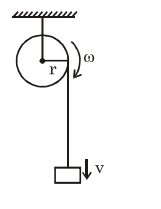# A cord is wound round the circumference of wheel of radius`
Question:

A cord is wound round the circumference of wheel of radius $\mathrm{r}$. The axis of the wheel is horizontal and the moment of inertia about it is I. A weight $\mathrm{mg}$ is attached to the cord at the end. The weight falls from rest. After falling through a distance ' $h$ ', the square of angular velocity of wheel will be :-

1. $\frac{2 m g h}{\mathrm{I}+2 \mathrm{mr}^{2}}$

2. $\frac{2 m g h}{I+m r^{2}}$

3. $2 \mathrm{gh}$

4. $\frac{2 g h}{I+m r^{2}}$

Correct Option: , 3

Solution:

$m g h=\frac{1}{2} I \omega^{2}+\frac{1}{2} m v^{2}$

$\mathrm{v}=\omega \mathrm{\omega r}$

$\mathrm{mgh}=\frac{1}{2} \mathrm{I} \omega^{2}+\frac{1}{2} \mathrm{~m} \omega^{2} \mathrm{r}^{2}$

$\frac{2 m g h}{\left(I+m r^{2}\right)}=\omega^{2}$